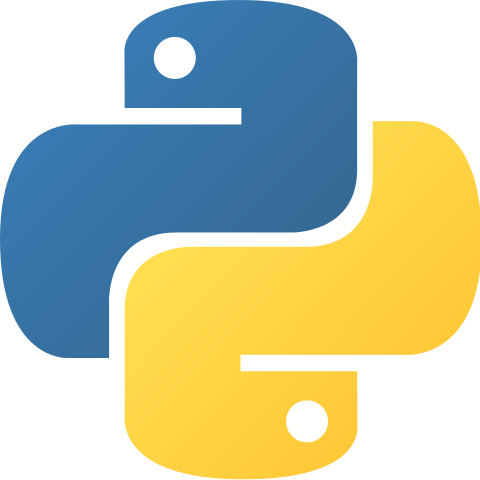# Count dots on any number of black and white cards as one input (using a loop)

Learning outcomesStudents will be able to:

## Requirement:

Write a program that asks the user to enter any number of black and white cards representing bits, all as one input ('B' for black and 'W' for white), and displays the total number of dots as the output.

## Testing examples:

Your program should display the outputs shown in this table for the given inputs provided:

Input Output
W
B
B
4
W 1
B 0
W
W
W
W
W
W
W
W
255

## Languages

Scratch#### What it should look like

Click on the green flag, enter the inputs provided in the “testing examples” to see the expected output of your program.

Recommended blocks
when green flag clicked

ask [Please enter a sequence of cards ('B' for black and 'W' for white):] and wait

if <(letter (index) of (cards)) = [W]> then
end

repeat (length of (cards))
end

say (total number of dots)
set [total number of dots v] to 

set [number of dots v] to 

set [index v] to (length of (cards))

set [total number of dots v] to ((total number of dots) + (number of dots))

set [number of dots v] to ((number of dots) * (2))

set [index v] to ((index) - (1))
Hints
• Make variables called:

• “total number of dots” and set its value to 0.
• “number of dots” and set its value to 1.
• “cards” and set its value to the string entered as the input. Check each letter of the input string “cards” (use a loop to iterate the number of letters in the input) starting from the last letter. If it’s ‘W’ add the corresponding number of dots (1 for the last letter, 2 for the second to last letter and so on) to the “total number of dots”. Display the “total number of dots” as the output.
• “index” and set its value to the number of letters in the input. Use this variable to access a letter at the “index” position in the string.
• You can access a letter at the specified position in a string by using the letter (1) of [world] block under “Operators”. For example: letter (1) of [world] //w

• In this challenge you need to access all the letters in user’s input and check to see if each of them is equal to B (black) or W (white).

• You can find how many letters a string has by using the length of [world] block unders “Operators”.

• The  if <> then block checks if the condition is true and then runs the blocks inside of the IF block. In this challenge you need to check if each letter of the input is equal to ‘W’ or “B’.

PythonBlock-based